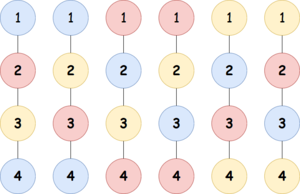Competitions

# Virus Tree 2

You are given a tree with n vertices and n1 edges. The vertices are numbered 1 to n, and the i-th edge connects vertices ai and bi.

You have coloring materials of k colors. For each vertex in the tree, you will choose one of the k colors to paint it, so that the following condition is satisfied:

• If the distance between two different vertices x and y is less than or equal to two, x and y have different colors.

How many ways are there to paint the tree? Find the count modulo 109 + 7.

#### Input

The first line contains two numbers n and k. Each of the next n - 1 lines contains two integers ai and bi.

#### Output

Print the number of ways to paint the tree, modulo 109 + 7.Time limit 1 second
Memory limit 128 MiB
Input example #1
4 3
1 2
2 3
3 4

Output example #1
6

Input example #2
5 4
1 2
1 3
1 4
4 5

Output example #2
48